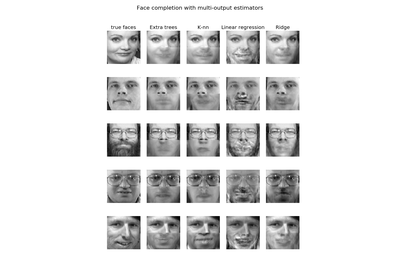# 3.2.4.1.9. `sklearn.linear_model`.RidgeCV¶

class `sklearn.linear_model.``RidgeCV`(alphas=(0.1, 1.0, 10.0), fit_intercept=True, normalize=False, scoring=None, cv=None, gcv_mode=None, store_cv_values=False)[source]

Ridge regression with built-in cross-validation.

By default, it performs Generalized Cross-Validation, which is a form of efficient Leave-One-Out cross-validation.

Read more in the User Guide.

Parameters: alphas : numpy array of shape [n_alphas] Array of alpha values to try. Regularization strength; must be a positive float. Regularization improves the conditioning of the problem and reduces the variance of the estimates. Larger values specify stronger regularization. Alpha corresponds to `C^-1` in other linear models such as LogisticRegression or LinearSVC. fit_intercept : boolean Whether to calculate the intercept for this model. If set to false, no intercept will be used in calculations (e.g. data is expected to be already centered). normalize : boolean, optional, default False If True, the regressors X will be normalized before regression. This parameter is ignored when fit_intercept is set to False. When the regressors are normalized, note that this makes the hyperparameters learnt more robust and almost independent of the number of samples. The same property is not valid for standardized data. However, if you wish to standardize, please use preprocessing.StandardScaler before calling fit on an estimator with normalize=False. scoring : string, callable or None, optional, default: None A string (see model evaluation documentation) or a scorer callable object / function with signature `scorer(estimator, X, y)`. cv : int, cross-validation generator or an iterable, optional Determines the cross-validation splitting strategy. Possible inputs for cv are: None, to use the efficient Leave-One-Out cross-validation integer, to specify the number of folds. An object to be used as a cross-validation generator. An iterable yielding train/test splits. For integer/None inputs, if `y` is binary or multiclass, `sklearn.model_selection.StratifiedKFold` is used, else, `sklearn.model_selection.KFold` is used. Refer User Guide for the various cross-validation strategies that can be used here. gcv_mode : {None, ‘auto’, ‘svd’, eigen’}, optional Flag indicating which strategy to use when performing Generalized Cross-Validation. Options are: ```'auto' : use svd if n_samples > n_features or when X is a sparse matrix, otherwise use eigen 'svd' : force computation via singular value decomposition of X (does not work for sparse matrices) 'eigen' : force computation via eigendecomposition of X^T X ``` The ‘auto’ mode is the default and is intended to pick the cheaper option of the two depending upon the shape and format of the training data. store_cv_values : boolean, default=False Flag indicating if the cross-validation values corresponding to each alpha should be stored in the cv_values_ attribute (see below). This flag is only compatible with cv=None (i.e. using Generalized Cross-Validation). cv_values_ : array, shape = [n_samples, n_alphas] or shape = [n_samples, n_targets, n_alphas], optional Cross-validation values for each alpha (if store_cv_values=True and cv=None). After fit() has been called, this attribute will contain the mean squared errors (by default) or the values of the {loss,score}_func function (if provided in the constructor). coef_ : array, shape = [n_features] or [n_targets, n_features] Weight vector(s). intercept_ : float | array, shape = (n_targets,) Independent term in decision function. Set to 0.0 if `fit_intercept = False`. alpha_ : float Estimated regularization parameter.

`Ridge`
Ridge regression
`RidgeClassifier`
Ridge classifier
`RidgeClassifierCV`
Ridge classifier with built-in cross validation

Methods

 `decision_function`(\*args, \*\*kwargs) DEPRECATED: and will be removed in 0.19. `fit`(X, y[, sample_weight]) Fit Ridge regression model `get_params`([deep]) Get parameters for this estimator. `predict`(X) Predict using the linear model `score`(X, y[, sample_weight]) Returns the coefficient of determination R^2 of the prediction. `set_params`(\*\*params) Set the parameters of this estimator.
`__init__`(alphas=(0.1, 1.0, 10.0), fit_intercept=True, normalize=False, scoring=None, cv=None, gcv_mode=None, store_cv_values=False)[source]
`decision_function`(*args, **kwargs)[source]

DEPRECATED: and will be removed in 0.19.

Decision function of the linear model.

Parameters: X : {array-like, sparse matrix}, shape = (n_samples, n_features) Samples. C : array, shape = (n_samples,) Returns predicted values.
`fit`(X, y, sample_weight=None)[source]

Fit Ridge regression model

Parameters: X : array-like, shape = [n_samples, n_features] Training data y : array-like, shape = [n_samples] or [n_samples, n_targets] Target values sample_weight : float or array-like of shape [n_samples] Sample weight self : Returns self.
`get_params`(deep=True)[source]

Get parameters for this estimator.

Parameters: deep : boolean, optional If True, will return the parameters for this estimator and contained subobjects that are estimators. params : mapping of string to any Parameter names mapped to their values.
`predict`(X)[source]

Predict using the linear model

Parameters: X : {array-like, sparse matrix}, shape = (n_samples, n_features) Samples. C : array, shape = (n_samples,) Returns predicted values.
`score`(X, y, sample_weight=None)[source]

Returns the coefficient of determination R^2 of the prediction.

The coefficient R^2 is defined as (1 - u/v), where u is the regression sum of squares ((y_true - y_pred) ** 2).sum() and v is the residual sum of squares ((y_true - y_true.mean()) ** 2).sum(). Best possible score is 1.0 and it can be negative (because the model can be arbitrarily worse). A constant model that always predicts the expected value of y, disregarding the input features, would get a R^2 score of 0.0.

Parameters: X : array-like, shape = (n_samples, n_features) Test samples. y : array-like, shape = (n_samples) or (n_samples, n_outputs) True values for X. sample_weight : array-like, shape = [n_samples], optional Sample weights. score : float R^2 of self.predict(X) wrt. y.
`set_params`(**params)[source]

Set the parameters of this estimator.

The method works on simple estimators as well as on nested objects (such as pipelines). The latter have parameters of the form `<component>__<parameter>` so that it’s possible to update each component of a nested object.

Returns: self :

## 3.2.4.1.9.1. Examples using `sklearn.linear_model.RidgeCV`¶Face completion with a multi-output estimators